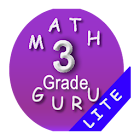All Android applications categories

All Android games categories205 7.2

7.2 Users
rating

## Screenshots

Description

Searching for third grade learning games ? You are at the right place.
Common Core Third grade mathematics tests (Infinite numbers - System generated questions and answers,test, questions are Different every time) for Third grade students.
This third grade learning games free contains Syllabus based on Common Core Standards. 3rd grade math games contains Levels to make your Kid Expert.

***Third grade maths APP is based on Common Core syllabus
3.OA Represent and solve problems (multiplication and Division)
3.OA Word Problems
3.OA Multiplication games
3.OA Division games
3. NF Fractions
3.OA Equations (properties and relationship)
3.OA Multiplication & Divsion
3.OA all four operations (Addition and Subtraction, Multiplication tables & Division)
3.NBT Numbers and operations in base ten
3.MD Measurement
3.MD Time
3.MD Represent and interpret data
3.G Geometry

Third grade maths common core math / worksheets / Third grade math activities:
Third class games / games for class third / third grade math activities: 3.OA Operations and Algebraic Thinking
Division problems for Third grade mathematics
free multiplication and division within 100 to solve word problems
properties of operations - Commutative property of multiplication , Associative property of multiplication , Distributive property
arithmetic patterns (including patterns in the addition table or multiplication table)
four operations, and identify and explain patterns in arithmetic

3rd grade math common core math 3.NBT Number and Operations in Base Ten with Worksheets / activities
place value for 3rd graders to understand properties of operations to perform multi-digit arithmetic in base ten
addition and subtraction - place value, properties of operations, and/or the relationship between addition and subtraction in base ten
Multiply one-digit whole numbers by multiples of 10.

Third grade Common Core math / games for class third / third grade math activities: 3.NF Number and Operations Fractions
fractions as numbers for 3rd grade students
fraction as a number on the number line
two fractions as equivalent (equal) if they are the same size
recognize and generate simple equivalent fractions
Express whole numbers as fraction
Compare two fractions same numerator /denominator

third class games / Worksheets - third grade math activities- common core math syllabus 3.MD Measurement and Data
measurement and estimation of intervals of time, liquid volumes, and masses of objects
time to the nearest minute and measure time intervals in minutes
measure and estimate liquid volumes and masses of objects using standard
geometric measurement: area and relate area to multiplication and to addition
third grade geometry : area of a rectangle,perimeter, Multiply side lengths

third class games / Worksheets / common core math games : 3.G Geometry
Solve problems related to reasoning with shapes and their attributes
Understand that shapes in different categories

With this third grade learning games free app, learn Mathematics wherever you are. 3rd grade math testing preparation helps your kid to become expert.

Tag : 3rd grade learning games free, games for 8 year olds, math games for 3rd graders free , grade 3 math, free kids learning games reading math for 2nd grade and third grade, games for class third, 3rd class games, maths practice app for class 3rd, math 3rd grade, kids math 6 years old, math class 3, third grade math games free.

refer our other apps : common core First Grade math , common core Second Grade math , common core Third Grade math , common core Fourth Grade math , common core Fifth Grade math , common core Sixth Grade math , common core Seventh grade math , Multiplication tables

from 205 reviews

"Great"

7.2